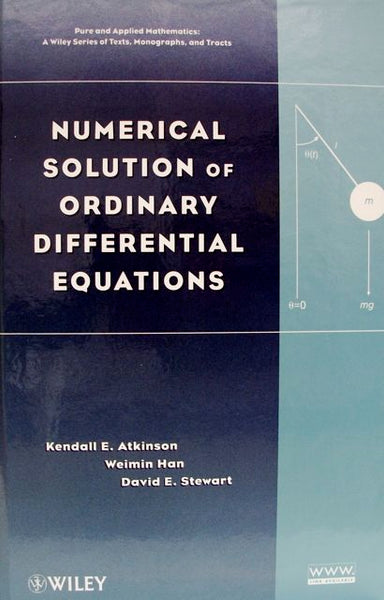# Numerical Solution Of Ordinary Differential Equations

: In stock

: Wiley

: 9780470042946

: Book

\$89.95

#### Details

Numerical Solution of Ordinary Differential Equations presents a complete and easy-to-follow introduction to classical topics in the numerical solution of ordinary differential equations. The book approach not only explains the presented mathematics, but also helps readers understand how these numerical methods are used to solve real-world problems.

• Isbn-13 Digit: 9780470042946
• Author-1: Kendall E. Atkinson
• Author-2: Weimin Han
• Author-3: David E. Stewart